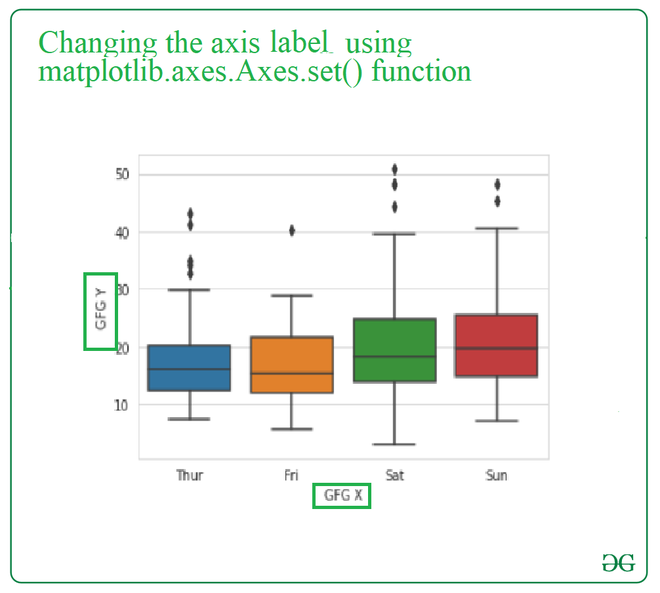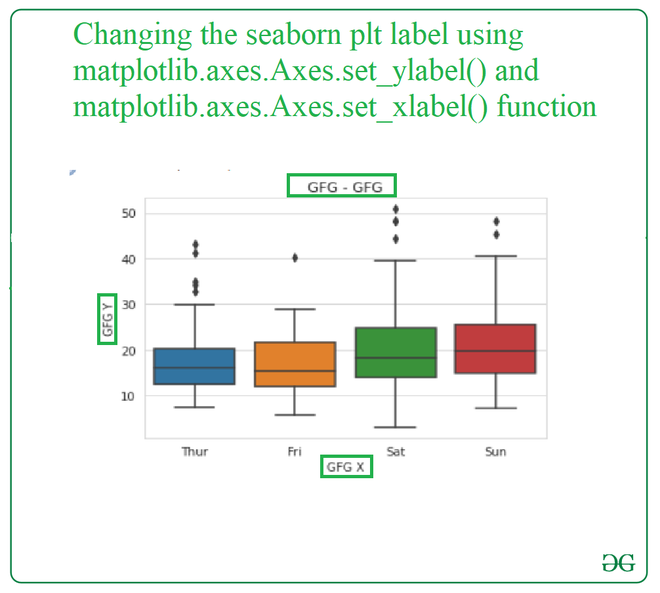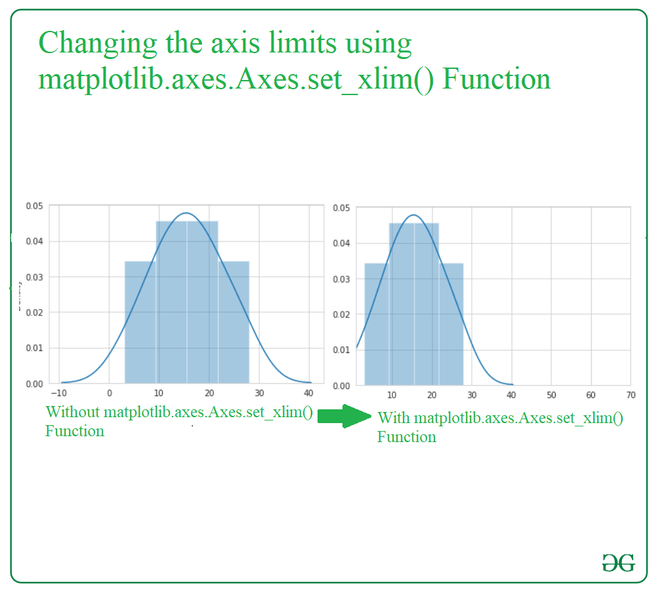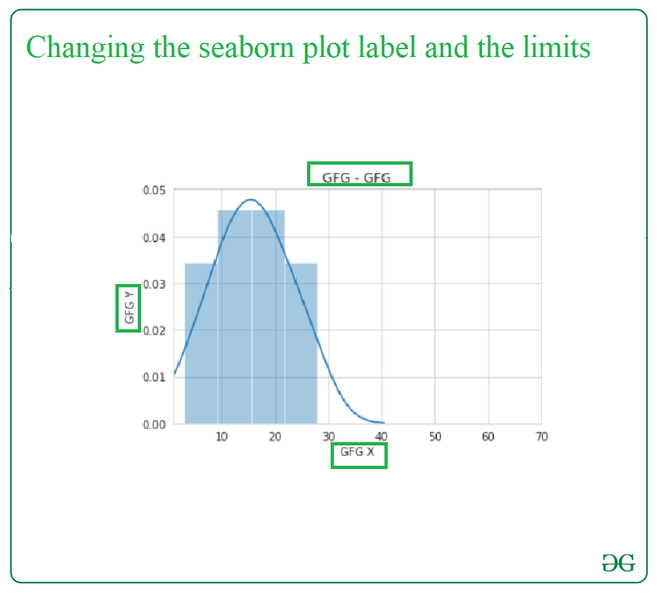# How to set axes labels & limits in a Seaborn plot?

• Last Updated : 14 Sep, 2021

In this article, we will learn How to set axes labels & limits in a Seaborn plot. Let’s discuss some concepts first.

• Axis is the region in the plot that contains the data space. The Axes contain two or three-axis(in case of 3D) objects which take care of the data limits.
• Axes Labels are the labels that describe the axes’ values in terms of meaning, units, and direction.
• Axes Limits are the limits to the axes’ values, which are used to filter for a required value on axes.

Here, In this article, the content goes from setting the axes labels, axes limits, and both at a time. In the end, you will be able to learn how to set axes labels & limits in a Seaborn plot.

Attention geek! Strengthen your foundations with the Python Programming Foundation Course and learn the basics.

To begin with, your interview preparations Enhance your Data Structures concepts with the Python DS Course. And to begin with your Machine Learning Journey, join the Machine Learning - Basic Level Course

## Set axes labels

Method 1: To set the axes label in the seaborn plot, we use matplotlib.axes.Axes.set() function from the matplotlib library of python.

Syntax: Axes.set(self, xlabel, ylabel, fontdict=None, labelpad=None, **kwargs)

Parameters:

• xlabel : str- The label text for the x-axis.
• ylabel : str- The label text for the y-axis.
• labelpad : scalar, optional, default: None
• **kwargs : Text properties

Returns: It will change the x-axis and y-axis labels.

Example: In this example, we will use single matplotlib.axes.Axes.set() function and change the label of the with a single call of this function, and we will pass both the xlabel and ylabel parameters in one go and this will change the user plot.

## Python3

 `# import seaborn``import` `seaborn as sns``sns.set_style(``"whitegrid"``)` `# import data``tips ``=` `sns.load_dataset(``"tips"``)` `# plot boxplot``gfg ``=` `sns.boxplot(x ``=``"day"``, y ``=``"total_bill"``, data ``=` `tips)` `# add label to the axis and label to the plot``gfg.``set``(xlabel ``=``"GFG X"``, ylabel ``=` `"GFG Y"``, title ``=``'some title'``)`

Output:Method 2: To set the axes label in the seaborn plot, we use matplotlib.axes.Axes.set_ylabel() and matplotlib.axes.Axes.set_xlabel() function from the matplotlib library of python.

Syntax:

Parameters: This method accepts the following parameters.

• xlabel : This parameter is the label text.
• labelpad : This parameter is the spacing in points from the axes bounding box including ticks and tick labels.

Returns:This method does not return any value.

Example: In this example, we will use matplotlib.axes.Axes.set_ylabel() and matplotlib.axes.Axes.set_xlabel() function separately and pass the name of the label as the parameter to change the label of the plot.

Input:

## Python3

 `# import seaborn``import` `seaborn as sns``sns.set_style(``"whitegrid"``)` `# load data``tips ``=` `sns.load_dataset(``"tips"``)` `# plot boxplot``gfg ``=` `sns.boxplot(x ``=``"day"``, y ``=``"total_bill"``, data ``=` `tips)``# This will add title to plot``gfg.set_title( ``"GFG - GFG"``)` `# This will add label to X-axis``gfg.set_xlabel( ``"GFG X"``)``# This will add label to Y-axis``gfg.set_ylabel( ``"GFG Y"``)`

Output:## Set axes limits

Functions for changing the limits:-

1. matplotlib.axes.Axes.set_xlim() Function: axes module of matplotlib library is used to set the x-axis view limits.
2. matplotlib.axes.Axes.set_ylim() Function: axes module of matplotlib library is used to set the y-axis view limits.

Syntax:

Axes.set_xlim(self, left=None, right=None, emit=True, auto=False, *, xmin=None, xmax=None)

Axes.set_ylim(self, bottom=None, top=None, emit=True, auto=False, *, ymin=None, ymax=None)

Parameters:

• bottom: This parameter is the bottom xlim/ylim in data coordinates
• top: This parameter is the top xlim/ylim in data coordinates
• emit: This parameter is used to notify observers of limit change.
• auto: This parameter is used to turn on autoscaling of the x-axis/y-axis.
• xmin, xmax, ymin, ymax: These parameters are equivalent to bottom and top and it is an error to pass both xmin/ymin and bottom or xmax/ymax and top.

Returns: bottom, top: This returns the new x-axis/y-axis limits in data coordinates.

Example:

## Python3

 `# import packages``import` `matplotlib.pyplot as plt``import` `seaborn as sns` `# create data``data ``=` `[``3``, ``7``, ``9``, ``11``, ``12``, ``14``, ``15``, ``16``, ``18``, ``19``, ``20``, ``23``, ``25``, ``28``]` `# plot distplot``fig, ax ``=` `plt.subplots()``sns.distplot(data, ax ``=` `ax)` `# change the limits of X-axis``ax.set_xlim(``1``, ``70``)``plt.show()`

Output:## Set axes labels and axes limits

In this particular example, we will be changing the label and the limit of the plot in a single code with the help of the suitable required functions of the python library.

## Python3

 `# import packages``import` `matplotlib.pyplot as plt``import` `seaborn as sns` `# create data``data ``=` `[``3``, ``7``, ``9``, ``11``, ``12``, ``14``, ``15``, ``16``, ``18``, ``19``, ``20``, ``23``, ``25``, ``28``]` `# plot distplot``fig, ax ``=` `plt.subplots()``sns.distplot(data, ax ``=` `ax)` `# This will change the limits of the x-axis``ax.set_xlim(``1``, ``70``)` `# This will add label to the X-axis``ax.set_xlabel( ``"GFG X"``)` `# This will add label to the Y-axis``ax.set_ylabel( ``"GFG Y"``)` `# This will add title to the plot``ax.set_title( ``"GFG - GFG"``) ``plt.show()`

Output:My Personal Notes arrow_drop_up